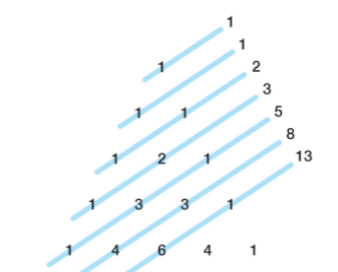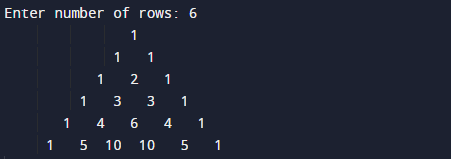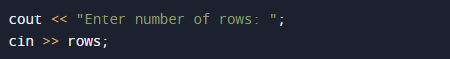# C++ Program to Print Pascal Triangle

In this tutorial you will learn about the C++ Program to Print Pascal Triangle and its application with practical example.

In this tutorial, we will learn to create a c++ program that  will create Pascal’s Triangle using c++ programming.

## Prerequisites

Before starting with this tutorial we assume that you are best aware of the following C++ programming topics.

• if-else Statements.
• Looping statement.
• Basic input/output.
• Operators.

## What Pascal Triangle?

The most interesting Numbers Pattern ,named after Blaise Pascal, a French Mathematician.Triangular arrangement of numbers that gives the coefficients in the expansion of any binomial expression.The triangle has some interesting patterns.Let us see an example, drawing parallel “shallow diagonals” and adding the numbers on each line together produces the Fibonacci series.

## C++ Program to Creating  Pascal Triangle.

In this program we will print  Pascal Triangle using nested  for loop. We would first declared and initialized the required variables. Then we will create  Pascal Triangle using below program.

## OutputIn the above program, we have first declared and initialized a set variables required in the program.• i & j= for  iteration.
• rows= for number of rows.
• coef= for Coefficient .
• space= for printing space.

Pascals triangle is a triangular array of the binomial coefficients.All values outside the triangle are zero (0). Program takes number from user to input total number of rows and using nested for loop to print pascal’s triangle.As shown belowHere  the inner loop creates the  space and the second inner loop computes the value of binomial coefficient, creates indentation space and prints the binomial coefficient for that particular column.

Let see the concept of creating a pascal triangle in step-by-step .

1 – Take value for number of rows to be printed in rows.
2 – Outer loop  runs for n times to print rows.
3 – Inner loop run for j to (rows – 1)
4 – That will print blank space ” “.
5 – ending the inner loop.
6 – Than another loop iterate form  j to i.
7 – Where we check condition=>
if (j == 0 || i == 0)
coef = 1
else
coef = coef*(i-j+1)/j.
8 – in each loop if condition satisfied we will print  coefficient value till the total number of rows.The program output is come  out as shown.

In this tutorial we have learn about the C++ Program to Print Pascal Triangle and its application with practical example. I hope you will like this tutorial.